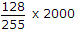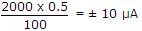# Electronics and Communication Engineering - Digital Electronics - Discussion

18.

An 8 bit DAC has a full scale output of 2 mA and full scale error of ± 0.5%. If input is 10000000 the range of outputs is

 [A]. 994 to 1014 μA [B]. 990 to 1020 μA [C]. 800 to 1200 μA [D]. none of the above

Explanation:

10000000 = 128, 11111111 = 255

If there is no error, output == 1004μA.

Maximum error =Hence range of output 994 to 1014 μA.

 Renuka said: (Sep 25, 2014) 2000 mentioned above indicates ?

 Sudha said: (Feb 23, 2015) 2 mA in μA.

 Sharon said: (Mar 12, 2015) Please give me clear explanation of above expression.

 Adi said: (Sep 5, 2016) Please give me the formula to be used here.

 Teju said: (Feb 10, 2017) 8bit DAC. Step size= Full scale/number of steps. Number of steps =255. =2ma/255=7.84m. Ideal output to input = step size*input range. 7.84 * 128 = 1004 Ma. Error = 0.5%*input range. =0.5%*2ma = 10ma.

 Prakash Kumar Saini said: (Mar 13, 2018) The step size is 2mA/255 = 7.84 μA. Since 10000000 = 12810, the ideal output should be 128 * 7.84 μA. The error can be as much as 0.5% * 2mA = 10μA. Thus, the actual output can deviate by this amount from the ideal, 1004μA , so the actual output can be anywhere from 994 to 1014 μA.

 Priyadarshini said: (Jul 30, 2018) How 128 as the input range here?

 A.R.Poomalai said: (Sep 29, 2018) @Priyadarshini. Convert this binary 10000000 to decimal you will get 128.

 Sujith K said: (Aug 15, 2019) But How this number of steps becomes 255, why not 256? So, 2^8=256.

 Akshit Jain said: (Apr 19, 2020) @Sujith K. Here we are considering maximum 8 bit number for the max range.